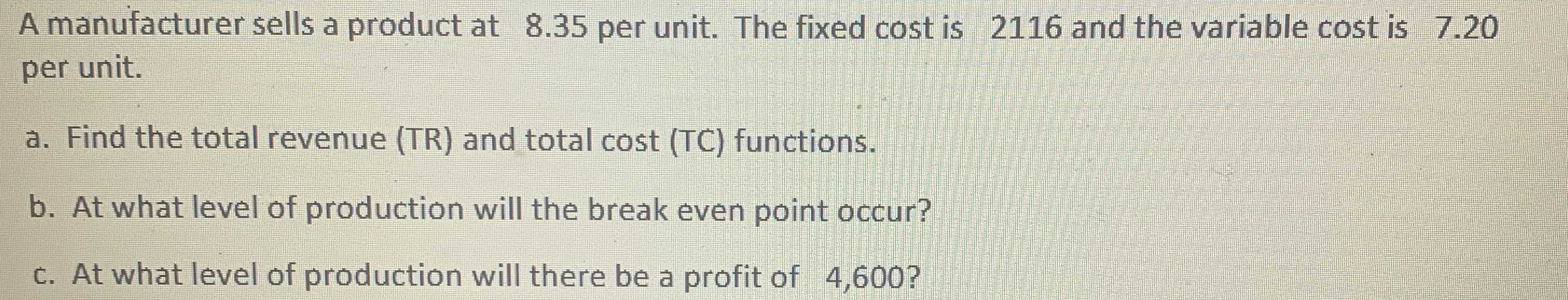### ¿Todavía tienes preguntas de matemáticas?

Pregunte a nuestros tutores expertos
Algebra
PreguntaA manufacturer sells a product at $$8.35$$ per unit. The fixed cost is $$2116$$ and the variable cost is $$7.20$$ per unit. a. Find the total revenue (TR) and total cost (TC) functions. b. At what level of production will the break even point occur? c. At what level of production will there be a profit of $$4,600 ?$$